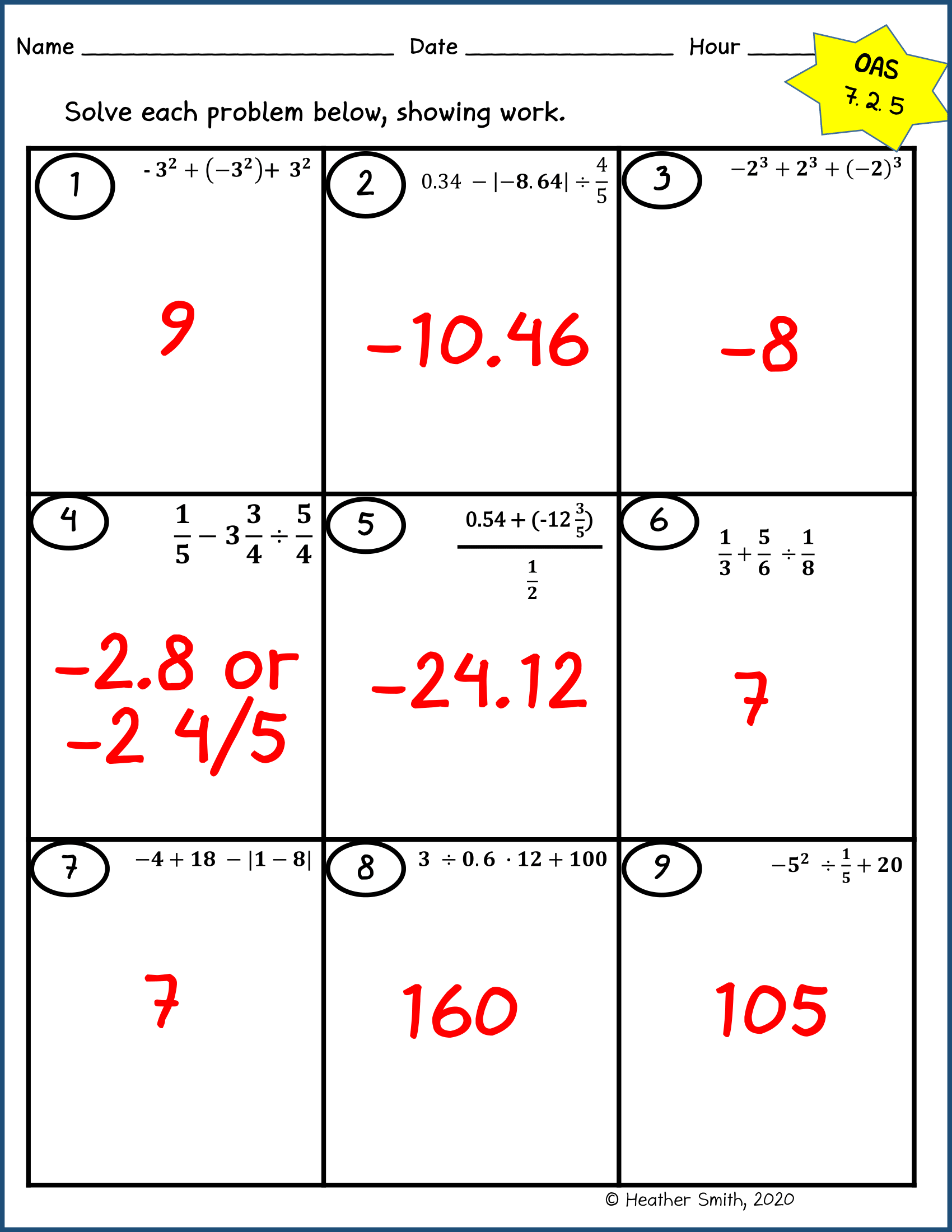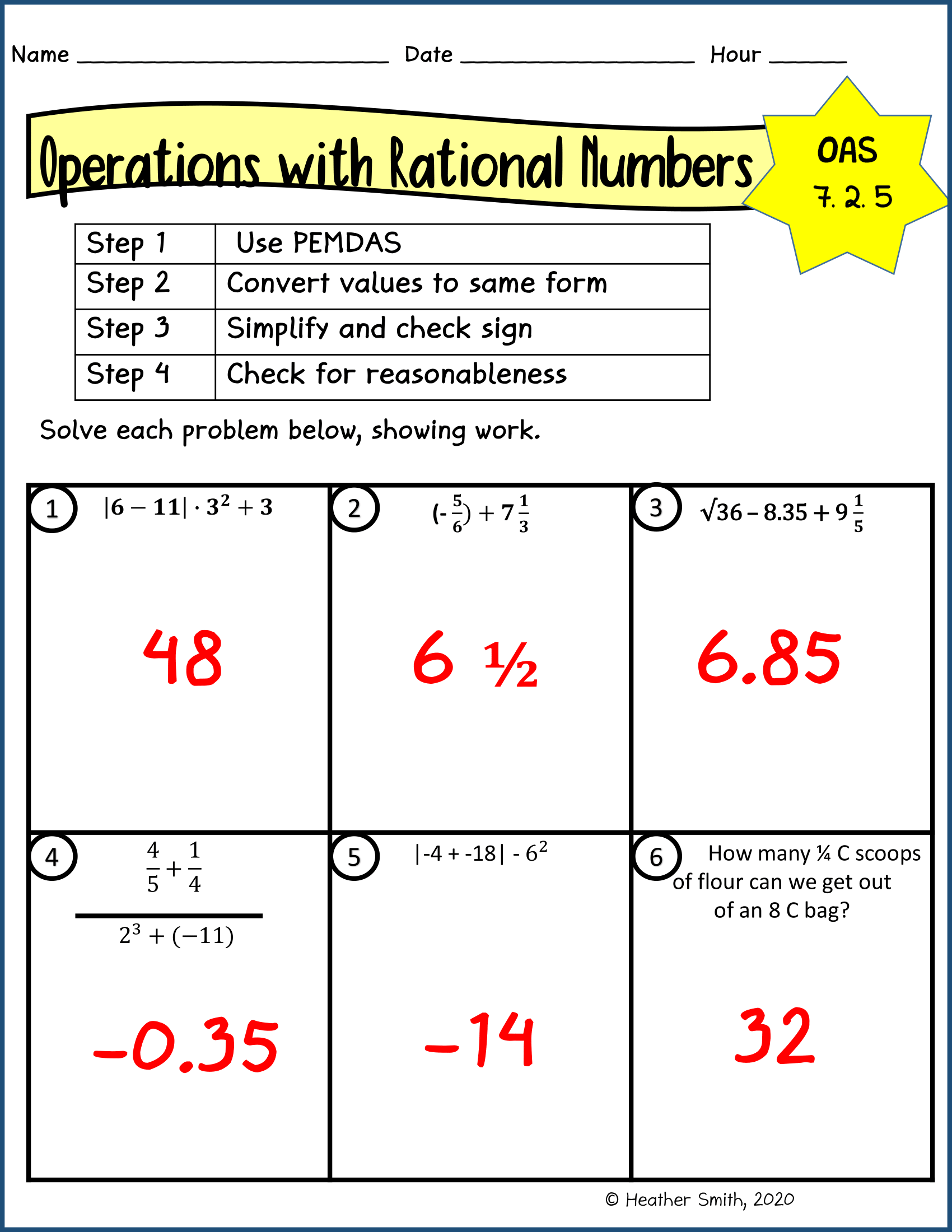# Grade 7 Rational Numbers Worksheets

## Grade 7 Maths Rational Numbers Multiple Choice Questions (MCQs)

1. Associative property is not followed in …………….
(a) whole numbers
(b) integers
(c) natural numbers
(d) none of these

2. ……………….. is the identity for the addition of rational numbers.
(a) 1
(b) 0
(c) -1
(d)$\frac{1}{2}$

3. ………………. is the multiplicative identity for rational numbers.
(a) 1
(b) 0
(c) -1
(d)$\frac{1}{2}$

4. The additive inverse of$\frac{7}{5}$ is:
(a) 1
(b) 0
(c) –$\frac{7}{5}$
(d)$\frac{7}{5}$

5. Zero has ……………. reciprocal.
(a) 1
(b) 2
(c) 3
(d) no

6. The numbers ……………. and ………….. are their own reciprocal.
(a) 1 and 0
(b) 1 and -1
(c) -1 and 0
(d) none of these

7. The reciprocal of -5 is ……………..
(a) 5
(b) 1
(c) –$\frac{1}{5}$
(d)$\frac{1}{5}$

8. The product of two rational numbers is always a …………………. .
(a) whole number
(b) integer
(c) natural number
(d) rational number

9. Simplify:$\frac{-4}{5} \times \frac{3}{7} \times \frac{15}{16} \times\left\{\frac{-14}{9}\right\}$
(a) 1
(b) 0
(c) 2
(d)$\frac{1}{2}$

10. What number should be added to$\frac{7}{12}$ to get$\frac{4}{15}$?
(a) –$\frac{9}{60}$
(b) –$\frac{11}{30}$
(c)$\frac{51}{60}$
(d)$\frac{1}{20}$

11. The reciprocal of a positive rational number is …………….. .
(a) negative
(b) positive
(c) zero
(d) none of these

12. Which of the rational numbers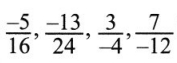is the smallest?
(a)$\frac{-5}{16}$
(b)$\frac{-13}{24}$
(c)$\frac{3}{-4}$
(d)$\frac{7}{-12}$

13. Rational number$\frac{3}{40}$ is equal to:
(a) 0.75
(b) 0.12
(c) 0.012
(d) 0.075

14. A rational number between 3 and 4 is:
(a)$\frac{3}{2}$
(b)$\frac{4}{3}$
(c)$\frac{7}{2}$
(d)$\frac{7}{4}$

15. Find x such that$\frac{-1}{5}$ =$\frac{8}{x}$
(a) -5
(b) -40
(c) any number
(d) none of these

16. Find x such that$\frac{-3}{8}$ and$\frac{x}{-24}$ are equivalent rational numbers.
(a) 3
(b) 9
(c) 8
(d) none of these

17. Find the value of (-6) ÷$\frac{2}{3}$.
(a) -9
(b) 9
(c) -4
(d) none of these

18. Rewrite the rational number$\frac{-18}{48}$ in the simplest form.
(a)$\frac{-9}{24}$
(b)$\frac{-3}{8}$
(c)$\frac{-3}{8}$
(d) none of these

19. Write the next rational number in the pattern: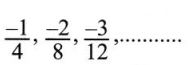(a)$\frac{-4}{15}$
(b)$\frac{-4}{16}$
(c)$\frac{-4}{20}$
(d) none of these

20. Write the next rational number in the pattern:(a)$\frac{12}{25}$
(b)$\frac{15}{25}$
(c)$\frac{-15}{25}$
(d) none of these

Grade 7 Maths Rational Numbers Fill in the boxes with the correct symbol out of >, < and =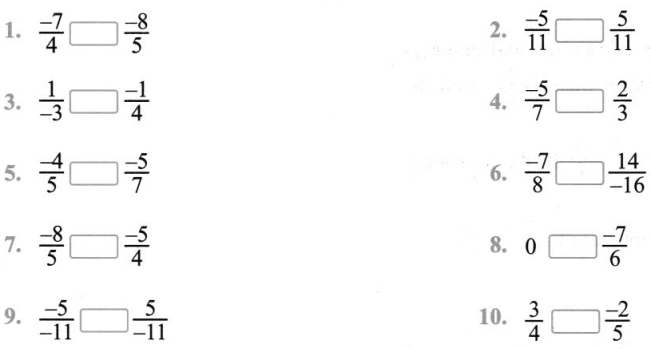Simplify:

1.$\frac{(-4)}{9} \times \frac{3}{5} \times \frac{(-9)}{10}$
2.$\left[\frac{2}{7}+\frac{3}{49}\right]\left[\frac{-7}{15}\right]$
3.$\frac{2}{3}+\frac{3}{4}+\frac{1}{12}$
4. (4.3 – 2.3) × 6.3
5.$\left[\frac{-28}{27}\right] \div\left[\frac{-5}{9}\right]$
6.$\frac{-4}{5}$ ÷ (-3)

1. Which is greater in the following numbers?
-3$\frac{2}{7}$, -3$\frac{4}{5}$
2. The product of two rational numbers is$\frac{-4}{5}$. If one of them is$\frac{8}{35}$, find the other.
3. Arrange$\frac{-7}{8}, \frac{-5}{6}, \frac{-3}{4}$ in the ascending order.
4. Find the reciprocal of$\frac{-7}{26}+\left(\frac{-11}{39}\right)$

(a) What should be added to$\frac{-5}{8}$ to get$\frac{2}{9}$
2. Jaspal donates$\left(\frac{1}{5}\right)^{t h}$ part of his monthly income and deposited$\left(\frac{1}{6}\right)^{t h}$ part in the bank and expenditure the remaining income.
3. Satpal walks$\frac{2}{3}$ km from a place P, towards east and then from there 1$\frac{5}{7}$ km towards west. Where will he be now from P?